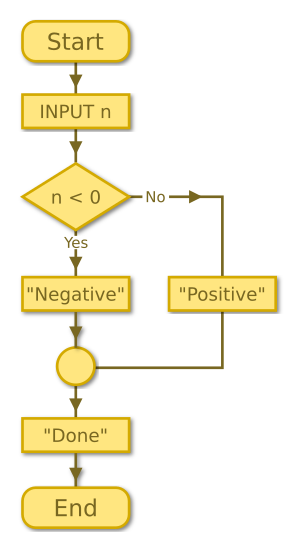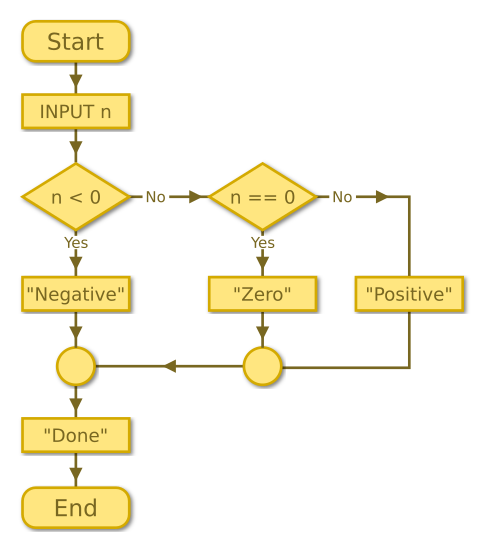# If statements

Martin McBride, 2017-02-03
Tags none
Categories none

We use selection to create code which only runs under the correct conditions.

The most common ways to do this are to use if statements, or to use switch statements. This section covers if statements.

## If statements

This code allows the user to input a number. It then prints a message only if the number is negative:

INT number = INPUT()
IF number < 0 THEN
PRINT("The number is negative")
ENDIF
PRINT("Done")


After inputting the number, the program uses an if statement to check if the number is less than 0.

If the condition is true (for example if the user input the number -3, which is less than 0), then the body of the if statement will run, printing the message.

If the condition is false (for example if the user input the number 5, which is not less than 0), then the body of the if statement will not run, so the message will not be printed.

{{% purple-note %}} The body of the if statement is the code between the IF ... THEN statement, and the ENDIF statement. This code only runs if the condition in the if statement is true. {{% /purple-note %}}

The final statement is outside the body of the if statement, so it always suns. It prints "Done".

Here is a short video which goes through the code, line by line:

## Conditions

There are 6 basic conditions:

• a < b - true if a is less than b.
• a > b - true if a is greater than b.
• a == b - true if a and b are exactly equal. Notice that we use a double equals sign, to avoid confusion with the assignment operator =.
• a <= b - true if a is less than or equal to b.
• a >= b - true if a is greater than or equal to b.
• a != b - true if a is not equal to b.

## If-else statements

An if-else statement allows us to run one section of code if the condition is true, or a different of code if the condition is not true. For example:

INT number = INPUT()
IF number < 0 THEN
PRINT("The number is negative")
ELSE
PRINT("The number is positive")
ENDIF
PRINT("Done")


{{% green-note %}} An if-else will always do one thing or the other. It will never do both, and it will never do nothing. {{% /green-note %}}

Here is a flowchart of the if-else code:## If-elseif statements

The only problem with the previous code is that it doesn't work in the case when number is 0. The if statement checks if number is less than zero (which it is not), and then says that 0 is positive.

Of course, 0 is neither negative nor positive, it is just 0. There are 3 possible cases, and we handle this using ELSEIF:

INT number = INPUT()
IF number < 0 THEN
PRINT("The number is negative")
ELSEIF number == 0 THEN
PRINT("The number is zero")
ELSE
PRINT("The number is positive")
ENDIF
PRINT("Done")


Here, we have added an extra check. Here is what the code does, in English:

{{% yellow-note %}} If the number is less than 0 print negative, otherwise if it is equal to 0 print zero, otherwise print positive. {{% /yellow-note %}}

You can add as many ELSEIF statements as you need. The code will execute the first statement which tests for true, and if none are true it will execute the ELSE statement.

{{% blue-note %}} When you use ELSEIF, exactly one statement will be executed, never more, never less. {{% /blue-note %}}

Here is a flowchart of the elseif code:## Nested if statements

Sometimes, the problem you are trying to solve doesn't fit neatly into the structure of if-elseif. For example, let's write some code to work out if a particular year is a leap year or not.

The rules are:

• If the year divides by 4 it is a leap year.
• Except if the year divides by 100, then it is not a leap year.
• Except id the year divides by 400, then it is a leap year.

So:

• 2017 isn't a leap year because it doesn't divide by 4.
• 2016 is a leap year because it does divide by 4.
• 1900 isn't a leap year because it divides by 100.
• 2000 is a leap year because it divides by 400.

Here is how we could code this as a nested for loop:

INT year = INPUT()
IF year divides by 4 THEN
IF year divides by 100 THEN
IF year divides by 400 THEN
PRINT("Leap year")
ELSE
PRINT("Not leap year")
ENDIF
ELSE
PRINT("Leap year")
ENDIF
ELSE
PRINT("Not leap year")
ENDIF
PRINT("Done")


{{% orange-note %}} You can use the modulo operator to check if the year divides by a particular number. {{% /orange-note %}}

## Comparing elseif and nested if

An elseif statement can always be converted to a nested if:

INT number = INPUT()
IF number < 0 THEN
PRINT("The number is negative")
ELSE
IF number == 0 THEN
PRINT("The number is zero")
ELSE
PRINT("The number is positive")
ENDIF
ENDIF
PRINT("Done")


It is better to use elseif in this case. The benefits are:

• the code is shorter with fewer levels of indentation (this can be important if you have several elseif clauses).
• It make the intent of the code clearer. You know for certain that exactly one branch will be executed.

You could also code the leap year case as an elseif:

INT year = INPUT()
IF year divides by 400 THEN
PRINT("Leap year")
ELSEIF year divides by 100 THEN
PRINT("Not leap year")
ELSEIF year divides by 4 THEN
PRINT("Leap year")
ELSE
PRINT("Not leap year")
ENDIF
PRINT("Done")


While this code is shorter than the original, some people might find it less clear because the logic of the actual algorithm has been rearranged quite a lot. In cases like this, you should do whatever you think is easier to read.